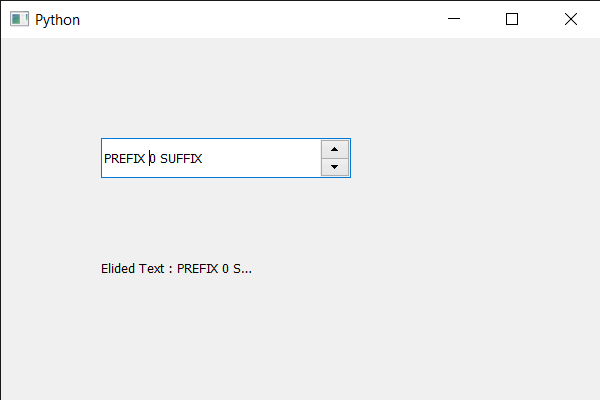# PyQt5 QSpinBox – How to get the elided text through font

In this article we will see how we can get the elided text of the spin box. If the string text is wider than width, an elided version of the string would be a string with “…” in it. Otherwise, original string will be displayed.

There are three mode parameters to get the elided text. The mode parameter specifies whether the text is elided on the left (e.g., “…tech”), in the middle (e.g., “Tr…ch”), or on the right (e.g., “Trol…”).

In order to do this we use `elidedText` method with the spin box’s QFontMetrics object.

Syntax : font_metrics.elidedText(text, Qt.ElideRight, 80)

Argument : It takes 3 argument, first is the spin box text, second is the elide mode and third is the width in pixel.

Return : It returns None

Below is the implementation

 `# importing libraries ` `from` `PyQt5.QtWidgets ``import` `*`  `from` `PyQt5 ``import` `QtCore, QtGui ` `from` `PyQt5.QtGui ``import` `*`  `from` `PyQt5.QtCore ``import` `*`  `import` `sys ` ` `  ` `  `class` `Window(QMainWindow): ` ` `  `    ``def` `__init__(``self``): ` `        ``super``().__init__() ` ` `  `        ``# setting title ` `        ``self``.setWindowTitle(``"Python "``) ` ` `  `        ``# setting geometry ` `        ``self``.setGeometry(``100``, ``100``, ``600``, ``400``) ` ` `  `        ``# calling method ` `        ``self``.UiComponents() ` ` `  `        ``# showing all the widgets ` `        ``self``.show() ` ` `  `        ``# method for widgets ` `    ``def` `UiComponents(``self``): ` `        ``# creating spin box ` `        ``self``.spin ``=` `QSpinBox(``self``) ` ` `  `        ``# setting geometry to spin box ` `        ``self``.spin.setGeometry(``100``, ``100``, ``250``, ``40``) ` ` `  `        ``# setting range to the spin box ` `        ``self``.spin.setRange(``0``, ``999999``) ` ` `  `        ``# setting prefix to spin ` `        ``self``.spin.setPrefix(``"PREFIX "``) ` ` `  `        ``# setting suffix to spin ` `        ``self``.spin.setSuffix(``" SUFFIX"``) ` ` `  `        ``# creating a label ` `        ``label ``=` `QLabel(``self``) ` ` `  `        ``# making label multi line ` `        ``label.setWordWrap(``True``) ` ` `  `        ``# setting geometry to the label ` `        ``label.setGeometry(``100``, ``200``, ``300``, ``60``) ` ` `  `        ``# getting font metrics ` `        ``f_metrics ``=` `self``.spin.fontMetrics() ` ` `  `        ``# text ` `        ``text ``=` `self``.spin.text() ` ` `  `        ``# getting elided text ` `        ``elided_text ``=` `f_metrics.elidedText(text, Qt.ElideRight, ``80``) ` ` `  `        ``# setting text to the label ` `        ``label.setText(``"Elided Text : "` `+` `str``(elided_text)) ` ` `  ` `  `# create pyqt5 app ` `App ``=` `QApplication(sys.argv) ` ` `  `# create the instance of our Window ` `window ``=` `Window() ` ` `  `# start the app ` `sys.exit(App.``exec``()) `

Output :Whether you're preparing for your first job interview or aiming to upskill in this ever-evolving tech landscape, GeeksforGeeks Courses are your key to success. We provide top-quality content at affordable prices, all geared towards accelerating your growth in a time-bound manner. Join the millions we've already empowered, and we're here to do the same for you. Don't miss out - check it out now!

Previous
Next